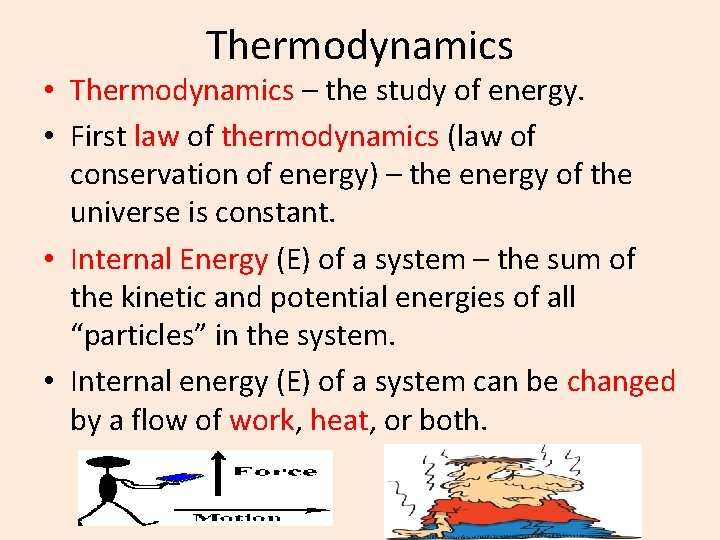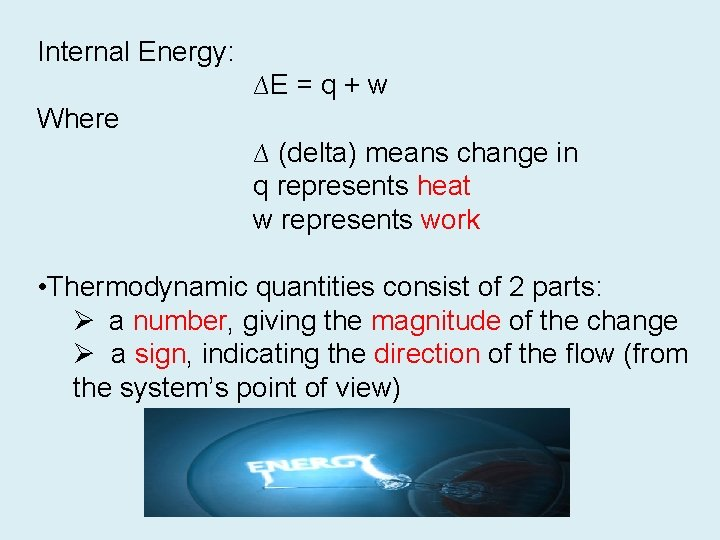# The Flow of Energy Thermodynamics Thermodynamics the study

• Slides: 8The Flow of EnergyThermodynamics • Thermodynamics – the study of energy. • First law of thermodynamics (law of conservation of energy) – the energy of the universe is constant. • Internal Energy (E) of a system – the sum of the kinetic and potential energies of all “particles” in the system. • Internal energy (E) of a system can be changed by a flow of work, heat, or both.Internal Energy: ∆E = q + w Where ∆ (delta) means change in q represents heat w represents work • Thermodynamic quantities consist of 2 parts: Ø a number, giving the magnitude of the change Ø a sign, indicating the direction of the flow (from the system’s point of view)q = heat flowing into or out of the system -q if energy is leaving to the surroundings (exothermic) +q if energy is entering from the surroundings (endothermic)w = work done by, or on, the system -w if work is done by the system on the surroundings (exothermic) +w if work is done on the system by the surroundings (endothermic)Measuring Energy Changes • Common units of energy: the calorie and the joule. • Calorie – amount of energy (heat) required to raise the temperature of 1 gram of water by 1 o. C. • The “calorie” with which you are familiar is used to measure the energy content of food and is actually a kilocalorie (1000 calories), written with a capital C (Calorie) to distinguish it from the calorie used in chemistry. • Joule (an SI unit) – defined in terms of the calorie: 1 calorie = 4. 184 joules Abbreviated 1 cal = 4. 184 J • You will need to be able to convert between the two units.Calculating Energy Requirements • Let’s say we want to determine how much energy is required to change the temperature of a substance, what calculation would be required? Energy (heat) required (q) = mass (g) x Specific heat capacity (J/g o. C) x ∆T (o. C) • Specific heat capacity – the amount of energy required to change the temperature of one gram of a substance by one Celsius degree. • Each substance has its own specific heat capacity.• Different substances respond differently to being heated. • For example, it takes 4. 184 J of energy to raise the temperature of 1 gram of water 1 o. C. • In contrast, the same amount of energy applied to 1 g of gold raises its temperature by approximately 32 o. C!! • Some substances require large amounts of energy to change their temperatures whereas others require small amounts. • The specific heat capacities for several substances are in the table. • Notice water has a very high specific heat. • This is why lakes and seas are so much slower to respond to cooling or heating than are the surrounding land masses.